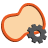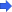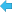Back to Adaptive Vision Library website

You are here: Start » Function Reference » Region » Region Features » RegionMoment# RegionMoment

Computes selected second-order moment of a region in regular and normalized ( divided by region area ) variant.

### Syntax

C++
C#

void avl::RegionMoment
(
const avl::Region& inRegion,
avl::RegionMomentType::Type inMomentType,
bool inCentral,
float& outMoment,
float& outNormMoment
)


### Parameters

Name Type Default DescriptioninRegion const Region& Input regioninMomentType RegionMomentType::TypeinCentral booloutMoment float&outNormMoment float&

### Description

The operation computes the mathematical features of a shape called moments. Those are sums computed as follows:

\begin{aligned} Moment_{2,0}(R) &= \sum_{p \in R} p_x^2 \\ Moment_{1,1}(R) &= \sum_{p \in R} p_x p_y \\ Moment_{0,2}(R) &= \sum_{p \in R} p_y^2 \end{aligned}

The summing is conducted over region pixels, while $$p_x$$ and $$p_y$$ denote, accordingly, x and y coordinate of a pixel.

When inCentral parameter is set, the region is shifted before computations, so that its mass center is at location (0,0).

### Hints

• If the input region is not guaranteed to be non-empty, precede this filter with SkipEmptyRegion.

### Errors

List of possible exceptions:

Error type Description
DomainError Empty region on input in RegionMoment.
DomainError Not supported moment type in RegionMoment.